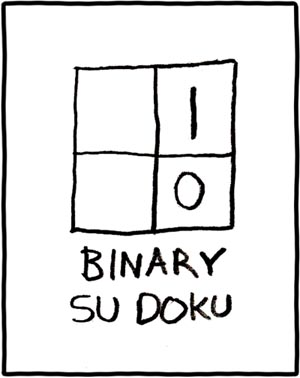# 74: Su Doku

 Su DokuTitle text: This one is from the Red Belt collection, of 'medium' difficulty

## Explanation

Su Doku (Japanese for "single number," and now usually written as "sudoku") is a type of number puzzle, in which the player must place digits in a matrix field in the correct arrangement, such that they do not repeat within given domains. The most common arrangement is a 9×9 grid subdivided into nine 3×3 grids, into which the nine non-zero digits of the normal decimal counting system must be inserted, with no digit being allowed to appear twice in a horizontal or vertical row or in each individual 3×3 grid. The number and combination of pre-filled squares determines the difficulty of the puzzle.

However, Randall presents a 2x2 binary sudoku puzzle which isn't subdivided. The joke is that the binary system has only two digits (0 and 1), and as a result binary sudoku puzzles would be trivially easy and thus pointless. The puzzle in the comic would be completed by filling 0 in the top-left and 1 in the bottom-left empty box. The only other possible grid would have the 0s and 1s swapped. This fulfills the criterion of having no repeated digits in any row, column or cell.

The title text appears to reference a series of published sudoku puzzle books called "Martial Arts Sudoku". The difficulty of each book is denoted by a martial arts belt color, with each color representing a certain skill level. A red belt is a rather high level, second only to the black belt. When applied to binary sudokus, a sudoku with one number given would be the most difficult one (though still trivial) and thus be a black belt. This sudoku has two numbers given, hence the medium red belt level.

## Transcript

[A square divided into 2×2 squares, the top-right one has an 1 in it, the bottom-right one has a 0, the two left ones are empty.]
Binary Su Dokuadd a comment! ⋅add a topic (use sparingly)! ⋅refresh comments!

# Discussion

If that puzzle is 4 (i.e. 2x2) domains of 1x1 cells or 1 domain of 4x4 cells then it's actually an impossible puzzle. Sudoku grids for 'n' symbols (ignoring some very interesting variants) need to be of n² cells in total with n cells in each direction, composed of n 'domains', each of n cells so as to contain one and only one of each symbol in use. That's 81 cells in a traditional 1-9 digit 9x9 format, being 3x3 array of 3x3 individual cells in typical Sudokus Sudokii Sudoka puzzles, but can be irregularly domained instead as long as the domains still have nine cells. In a 12-digit that's often 3x4 cells in each domain, arrayed 4x3 (or 4x3 arrayed 3x4) to make a 12x12 full grid but can be 2x6 6x2s (or <=>) or of irregular, but still equally-sized, subdivisions. ("Killer" variations typically augment the row, column and domain parities with a 'fourth dimension' of unequally-sized irregular domains (no larger than any other domain, containing a maximum of one of each digit, but possibly zero of some) labelled as having a stated sum total within (more than or equal to m*(m-1)/2 for m cells in that given sum-zone, assuming the lowest digit is 1, and less than or equal to (n*(n-1)/2)-((n-m)*(n-m-1)/2), if n digits are being used as unique symbols throughout the whole grid), but that's generally in leiu of all pre-existing clue digits, using Kakuro-like calculations to break ground on the puzzle's answer.)

Realistically, therefore, the comic must be 1x2 domains of 2x1 cells. Or the other way round. Although it's not obvious from the line-weighting which it might be. As each subdivision is the same as the row-grouping or column-grouping it could effectively be just a 'simpler' puzzle that abandons or considers redundant domains other than the basic rows and columns, given that each possible domain-type would be congruent with one or other of the two implicit groupings. However, it definitely could not be an "X" variant of the puzzle type (repetition dissallowed across the major diagonals, as well as across rows and columns), otherwise it reverts to being impossible again...

However, none of what I've just said is particularly entertaining, so please feel free to ignore it and instead try the following Unary Sudoku.... (Hint: its major diagonals are also valid domains to solve!)

178.98.31.27 15:07, 24 June 2013 (UTC)

## Major update

I think we even can discus PIxPI grids here at discussion page, but the explain should be simple as possible. Please help on that bad remaining language. AND: Since Randall is from the US we have AE (American English) here.--Dgbrt (talk) 21:19, 1 July 2013 (UTC)

And Su Doku is only 1 to 9 - thanks for help--Dgbrt (talk) 22:14, 9 July 2013 (UTC)

I think this description of the alt text is a little inadequate. The reason the puzzle is 'medium difficulty' is because any given puzzle in a binary sudoku is going to be essentially the same... you'll either have a one and a zero, two ones, or two zeroes since those are the only ways to ensure a unique solution. So all puzzles have the same difficulty which is why it is 'medium'.--108.162.238.162 08:28, 28 July 2015 (UTC)

I don't follow your sentence "you'll either have a one and a zero, two ones, or two zeroes", please can you try and explain more clearly. Essentially though, there are two possible solutions to the puzzle:
 0 1 or alternatively 1 0 1 0 0 1
The only thing which can make the difficultly change is how many cells are pre-filled. If 0 are filled then either both solutions are correct, or it is impossible to know which solution is correct. If 1 cell is filled, then it is easy to complete the rest of the grid. If 2 are filled, then it is even easier to complete, and easier again with 3 filled. --Pudder (talk) 11:01, 28 July 2015 (UTC)